# NCERT Solutions for Class 8 Math Chapter 3 - Understanding Quadrilaterals

##### Question 1:

Take any quadrilateral, say ABCD shown in figure. Divide it into two triangle, by drawing a diagonal. You get six angles 1, 2, 3, 4, 5 and 6.
Use the angle sum property of a triangle and argue how the sum of the measures of $\angle$A, $\angle$B, $\angle$C and $\angle$D amounts to 180$°$ + 180$°$ = 360$°$.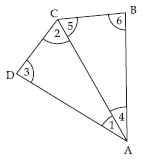$\angle$A + $\angle$B + $\angle$C + $\angle$D
= ($\angle$1 + $\angle$4) + $\angle$6 + ($\angle$5 + $\angle$2) + $\angle$3
= ($\angle$3 + $\angle$1 + $\angle$2) + ($\angle$4 + $\angle$6 + $\angle$5)
= 180$°$ + 180$°$ = 360$°$ [By angle sum property of a triangle]

##### Question 2:

Take four congruent card-board copies of any quadrilateral ABCD, with angles as shown fig (i). Arrange the copies as shown in the figure, where angles $\angle$1 + $\angle$2 + $\angle$3 + $\angle$4 meet at a point as shown in fig. (ii)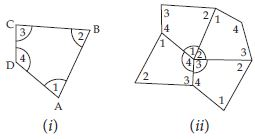What can you say about the sum of the angles $\angle$1, $\angle$2, $\angle$3 and $\angle$4?

We know that, the sum of the angles $\angle$1, $\angle$2, $\angle$3 and $\angle$4 is 360$°$.
The sum of the measure of the four angles of a quadrilateral is 360$°$.
[Note: We denote the angles by $\angle$1, $\angle$2, $\angle$3, etc., and their respective measures by m$\angle$1, m$\angle$2, m$\angle$3, etc.]
The sum of the measures of the four angles of a quadrilateral is 360$°$.

##### Question 3:

Take any quadrilateral ABCD shown in figure. Let P be any point in its interior. Join P to vertices A, B, C and D. In the figure, consider $∆$PAB. From this we see x = 180$°$ – m$\angle$2 – m$\angle$3; similarly from $∆$PBC, y = 180$°$ – m$\angle$4 – m$\angle$5, from $∆$PCD, z = 180$°$ – m$\angle$6 – m$\angle$7 and from $∆$PDA, w = 180$°$ – m$\angle$8 – m$\angle$1.
Use this to find the total measure m$\angle$1 + m$\angle$2 +… + m$\angle$8, does it help to arrive at the result?
Remember: $\angle$x + $\angle$y + $\angle$z + $\angle$w = 360$°$.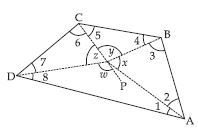Given that
x = 180$°$ – m$\angle$2 – m$\angle$3 ...(i)
y = 180$°$ – m$\angle$4 – m$\angle$5 ...(ii)
z = 180$°$ – m$\angle$6 – m$\angle$7 ...(iii)
w = 180$°$ – m$\angle$8 – m$\angle$1 ...(iv)
Adding equations (i), (ii), (iii) and (iv), we get
x + y + z + w = 720$°$ – ($\angle$1 + $\angle$2 + $\angle$3 + $\angle$4 + $\angle$5 + $\angle$6 + $\angle$7 + $\angle$8)
$⇒$ 360$°$ = 720$°$ – ($\angle$1 + $\angle$2 + $\angle$3 + $\angle$4 + $\angle$5 + $\angle$6 + $\angle$7 + $\angle$8)
$⇒$ $\angle$1 + $\angle$2 + $\angle$3 + $\angle$4 + $\angle$5 + $\angle$6 + $\angle$7 + $\angle$8 = 720$°$ – 360$°$ = 360$°$
$\therefore$ $\angle$A + $\angle$B + $\angle$C + $\angle$D = 360$°$
$\therefore$ The sum of the measures of the four angles of a quadrilateral is 360$°$.

##### Question 4:

Consider quadrilateral ABCD. Split it into two triangles and find the sum of the interior angles shown in figure.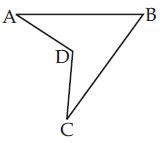Divide the quadrilateral ABCD into two triangles ABD and CBD.
In $∆$ABD, $\angle$A + $\angle$ABD + $\angle$ADB = 180$°$ ...(i)
and in $∆$BDC, $\angle$C + $\angle$CDB + $\angle$DBC = 180$°$ ...(ii)
Adding quations (i) and (ii), we get
$\angle$A + $\angle$ADB + $\angle$ABD + $\angle$C + $\angle$CDB + $\angle$DBC =
180$°$ + 180$°$ = 360$°$
$⇒$ $\angle$A + $\angle$ADB + $\angle$CDB + $\angle$C + ($\angle$CBD +
$\angle$DBA) = 360$°$
$⇒$ $\angle$A + $\angle$B + $\angle$C + $\angle$D = 360$°$##### Question 5:

Given here are some figures: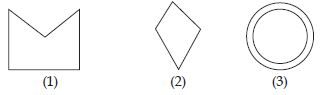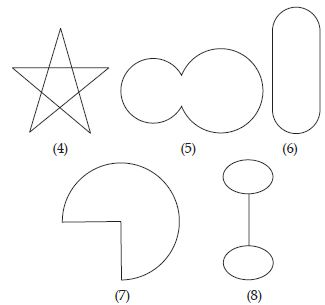Classify each of them on the basis of the
following:
(a) Simple curve
(b) Simple closed curve
(c) Polygon
(d) Convex polygon
(e) Concave polygon

(a) (1), (2), (5), (6), (7)
(b) (1), (2), (5), (6), (7)
(c) (1), (2), (4)
(d) (2)
(e) (1), (4)

##### Question 6:

How many diagonals does each of the following have:
(b) A regular hexagon
(c) A triangle

(a) A convex quadrilateral has 2 diagonals.
(b) A regular hexagon has 9 diagonals.
(c) A triangle has no diagonal.

##### Question 7:

What is the sum of the measures of the angles of a convex quadrilateral? Will this property hold if the quadrilateral is not convex?

360$°$, Yes. (make a non-convex quadrilateral and try!)

##### Question 8:

Examine the table (Each figure is divided into triangles and the sum of the angles deduced from that?What can you say about the angle sum of a convex polygon with number of sides?

(a) 7     (b) 8     (c) 10     (d) n

(a) The angle sum of a convex polygon with 7 sides is given by:
(7 – 2) × 180$°$ = 5 × 180$°$ = 900$°$
(b) The angle sum of a convex polygon with 8 sides is given by:
(8 – 2) × 180$°$ = 6 × 180$°$ = 1080$°$
(c) The angle sum of a convex polygon with 10 sides is given by:
(10 – 2) × 180$°$ = 8 × 180$°$ = 1440$°$
(d) The angle sum of a convex polygon with n sides is given by:
(n – 2) × 180$°$.

##### Question 9:

What is a regular polygon?
State the name of a regular polygon of:
(i) 3 sides (ii) 4 sides (iii) 6 sides.

Regular polygon: A regular polygon is both “equiangular” and “equilateral”.
(i) A regular polygon having 3 sides is called equilateral triangle.
(ii) A regular polygon having 4 sides is called square.
(iii) A regular polygon having 6 sides is called regular hexagon.

##### Question 10:

Find the angle measure x in the following figures: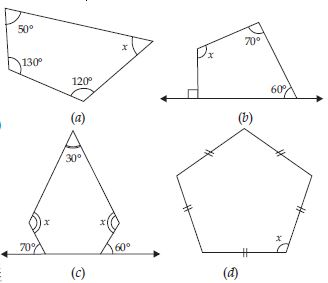(a) 50$°$ + 130$°$ + 120$°$ + x = 360$°$
300$°$ + x = 360$°$
$⇒$ x = 360$°$ – 300$°$ = 60$°$
(b) 90$°$ + 60$°$ + 70$°$ + x = 360$°$
$⇒$ 220$°$ + x = 360$°$
$⇒$ x = 360$°$ – 220$°$ = 140$°$
(c) Figure (c) has five sides:
$\therefore$ its angle sum = (5 – 2) × 180$°$
= 3 × 180$°$ = 540$°$
Also, exterior angles 70$°$ and 60$°$ are
given.
$\therefore$ Corresponding interior angles are (180$°$
– 70$°$) = 110$°$ and (180$°$ – 60$°$) = 120$°$ respectively.
$\therefore$ 110$°$ + 120$°$ + x + 30$°$ + x = 540$°$
260$°$ + 2x = 540$°$
$⇒$ 2x = 540$°$ – 260$°$
$⇒$ 2x = 280$°$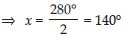Figure (d) is a regular pentagon.
$\therefore$ Its angle sum = (5 – 2) × 180$°$
= 3 × 180$°$ = 540$°$
$\therefore$ x + x + x + x + x = 540$°$
$⇒$ 5x = 540$°$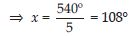##### Question 11:In the given figure:
Exterior angle x = (180$°$ – 90$°$) = 90$°$
Exterior angle z = (180$°$ – 30$°$) = 150$°$
As sum of interior angles of a triangle is 180$°$.
$\therefore$ 90$°$ + 30$°$ + p = 180$°$
120$°$ + p = 180$°$
$⇒$ p = 180$°$ – 120$°$ = 60$°$
Exterior angle y = (180$°$ – 60$°$) = 120$°$
$\therefore$ x + y + z = 90$°$ + 150$°$ + 120$°$
= 360$°$.

##### Question 12:In the given figure: Exterior angle x = (180$°$ – 120$°$) = 60$°$
Exterior angle y = (180$°$ – 80$°$) = 100$°$
Exterior angle z = (180$°$ – 60$°$) = 120$°$
As sum of interior angles of a quadrilateral is
360$°$.
$\therefore$ 120$°$ + 80$°$ + 60$°$ + q = 360$°$
$\therefore$ 260$°$ + q = 360$°$
$⇒$ q = 360$°$ – 260$°$
$⇒$ q = 100$°$
$\therefore$ Exterior angle w = (180$°$ – 100$°$)
= 80$°$
$\therefore$ x + y + z + w = 60$°$ + 100$°$ + 120$°$ + 80$°$
= 360$°$

##### Question 13:

Take a regular hexagon.
What is the sum of measure of its exterior
angles x, y, z, p, q, r?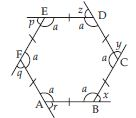Let ABCDEF is a regular hexagon having each side equal.As the sum of the measures of the external angles of any polygon is 360$°$.
$\therefore$ x + y + z + p + q + r = 360$°$

##### Question 14:

Take a regular hexagon.
Is x = y = z = p = q = r? Why?Yes, x = y = z = p = q = r, because the hexagon is regular.

##### Question 15:

Take a regular hexagon.
What is the measure of each exterior angle?The measure of each exterior angle is given
by x + x + x + x + x + x = 360$°$
$⇒$ 6x = 360$°$
$⇒$ x = 60$°$

##### Question 16:

Take a regular hexagon.
What is the measure of each interior angle?Measure of each interior angle
= 180$°$– 60$°$ = 120$°$

##### Question 17:

Take a regular octagon.
What is the measure of each exterior and interior angle?

The octagon being regular having 8 sides.
$\therefore$ All the exterior angles have equal measure,
say x.
$\therefore$ 8x = 360$°$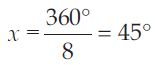$\therefore$ Measure of each exterior angle = 45$°$
Measure of each interior angle = 180$°$ – 45$°$
= 135$°$

##### Question 18:

Take a regular 20-gon.
What is the measure of each exterior and interior angle?

The polygon being regular having 20 sides.
$\therefore$ All the exterior angles have equal measure, say x.
$\therefore$ 20 x = 360$°$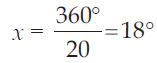$\therefore$ Measure of each exterior angle = 180$°$
Measure of each interior angle = 180$°$ – 18$°$
= 162$°$

##### Question 19:

Find x in the following figures.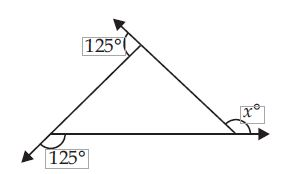As the sum of the measures of the external angles
of any polygon is 360$°$.
$\therefore$ 125$°$ + 125$°$ + x = 360$°$
250$°$ + x = 360$°$
$⇒$ x = 360$°$ – 250$°$ = 110$°$

##### Question 20:

Find x in the following figures.As the sum of the measures of the external angles
of any polygon is 360$°$.
$\therefore$ x + 90$°$ + 60$°$ + 90$°$ + 70$°$ = 360$°$
$⇒$ x + 310$°$ = 360$°$
$⇒$ x = 360$°$ – 310$°$
$⇒$ x = 50$°$

##### Question 21:

Find the measure of each exterior angle of a regular polygon of 9 sides.

The polygon being regular having 9 sides.
$\therefore$ All the exterior angles have equal measure,
say x.
$\therefore$ 9x = 360$°$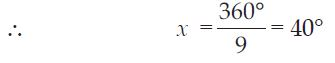$\therefore$ Measure of each exterior angle = 40$°$

##### Question 22:

Find the measure of each exterior angle of a regular polygon of 15 sides.

The polygon being regular having 15 sides.
$\therefore$ All the exterior angles have equal measure, say x.
$\therefore$ 15x = 360$°$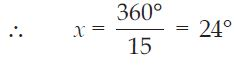$\therefore$ Measure of each exterior angle = 24$°$

##### Question 23:

How many sides does a regular polygon have if the measure of an exterior angle is 24$°$?

Total measure of all exterior angles = 360$°$
Measure of each exterior angle = 24$°$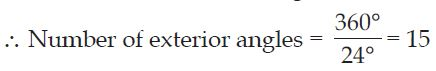$\therefore$ The polygon has 15 sides.

##### Question 24:

How many sides does a regular polygon have if each of its interior angles is 165$°$?

Measure of each interior angle = 165$°$
$\therefore$ Measure of each exterior angle
= 180$°$ – 165$°$
= 15$°$
Total measure of all exterior angles = 360$°$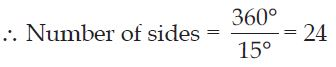$\therefore$ The polygon has 24 sides.

##### Question 25:

Is it possible to have a regular polygon with measure of each exterior angle as 22$°$?

No; since 22 is not a divisor of 360.

##### Question 26:

Can 22$°$ be an interior angle of a regular polygon? Why?

No; because each exterior angle is (180$°$ – 22$°$)
= 158$°$, which is not a divisor of 360$°$.

##### Question 27:

What is the minimum interior angle possible for a regular polygon? Why?

The equilateral triangle being a regular polygon of 3 sides has the least measure of an interior angle is equal to 60$°$.

##### Question 28:

What is the maximum exterior angle possible for a regular polygon?

The equilateral triangle being a regular polygon of 3 sides has the least measure of an interior angle is equal to 60$°$.
The greatest exterior angle of an equilateral triangle can be (180$°$ – 60$°$) = 120$°$.

##### Question 29:

Take identical cut-outs of congruent triangles of sides 3 cm, 4 cm, 5 cm. Arrange them as shown figure.
You get a trapezium. (Check it!) Which are the parallel sides here? Should the non-parallel sides be equal?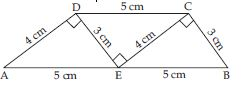You can get two more trapeziums using the same set of triangles. Find them out and discuss their shapes.

DC = AE = 5 cm
and AD = EC = 4 cm
DC || AE $⇒$ AD || CE
$\therefore$ DCEA is a parallelogram.
$\therefore$ ABCD is a trapezium. Its parallel sides are AB and DC. Non-parallel sides are AD and CB.
Two more examples of trapeziums using the same set of triangles.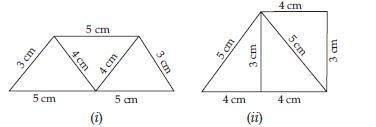##### Question 30:

In a parallelogram m$\angle$R = m$\angle$N = 70$°$, find m$\angle$1 and m$\angle$G.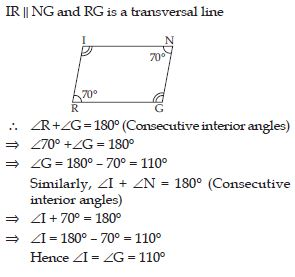##### Question 31: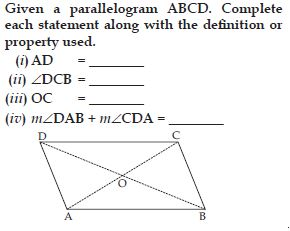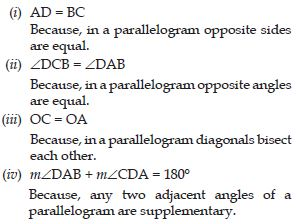##### Question 32:

Consider the following parallelograms. Find the values of the unknown x, y, z.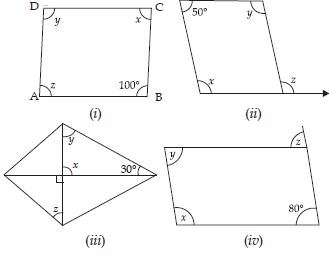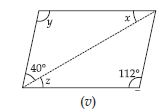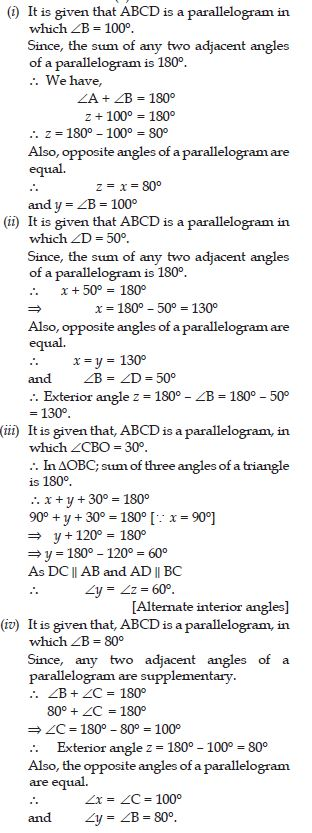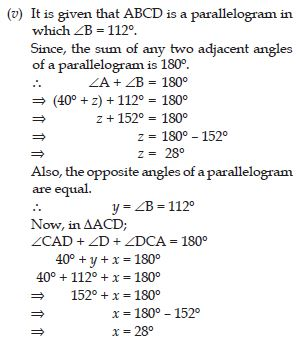##### Question 33:

Can a quadrilateral ABCD be a parallelogramif
(i) $\angle$D + $\angle$B = 180$°$
(ii) AB = DC = 8 cm, AD = 4 cm and BC = 4.4 cm?
(iii) $\angle$A = 70$°$ and $\angle$C = 65$°$?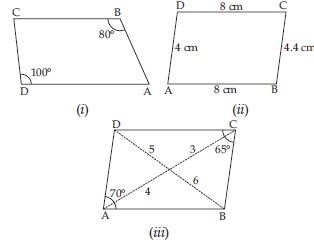Fig. (i) is not a parallelogram, because, the opposite angles i.e., $\angle$C and $\angle$A are not equal. Fig. (ii) is not a parallelogram, because, the opposite sides i.e, AB and CD and BC and DA are not equal.
Fig. (iii) is also not a parallelogram, because, the diagonals of a parallelogram bisect each other and here it is not so and $\angle$A and $\angle$C are not equal.

##### Question 34:

Draw a rough figure of a quadrilateral that is not a parallelogram but has exactly two opposite angles of equal measure.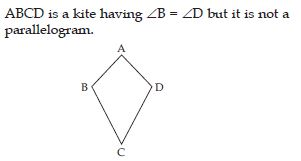##### Question 35:

The measures of two adjacent angles of a parallelogram are in the ratio 3 : 2. Find the measure of each of the angles of the parallelogram.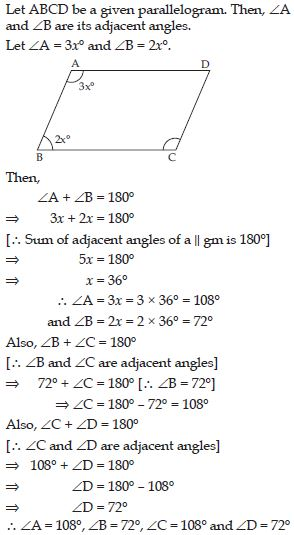##### Question 36:

Two adjacent angles of a parallelogram have equal measure. Find the measure of each of the angles of the parallelogram.

It is given that, ABCD is a parallelogram in which two adjacent angles $\angle$A and $\angle$B have equal measure, say x.
$\therefore$ m$\angle$A = x$°$ and m$\angle$B = x$°$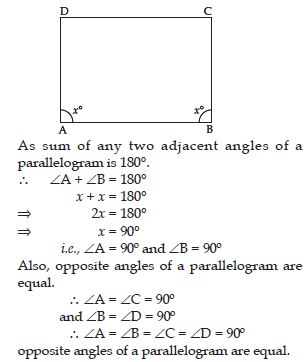##### Question 37:

The adjacent figure HOPE is a parallelogram.
Find the angle measures x, y and z. State the properties you use to find them.##### Question 38:

The following figures GUNS and RUNS are parallelograms. Find x and y. (Lengths are in cm.)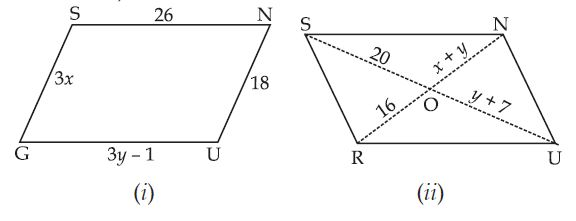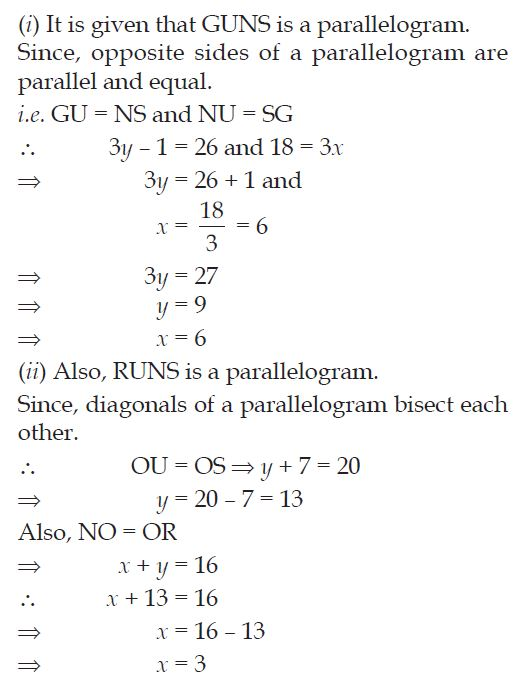##### Question 39: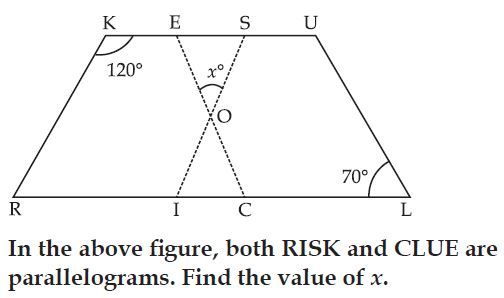It is given that RISK and CLUE are parallelograms.
$\therefore$ In parallelogram RISK;
$\angle$RKS + $\angle$ISK = 180$°$ [Because, sum of any two
adjacent angles of a parallelogram is 180$°$.]
$\therefore$ 120$°$ + $\angle$ISK = 180$°$
$⇒$ $\angle$ISK = 180$°$ – 120$°$ = 60$°$
Also, in parallelogram CLUE,
$\angle$CLU = $\angle$CEU = 70$°$
[Because, opposite angles of a parallelogram are equal]
$\therefore$ In $∆$OES; sum of three angles is equal to 180$°$.
$\therefore$ $\angle$OES + $\angle$ESO + x = 180$°$
[$\therefore$ $\angle$OES = $\angle$CEU and $\angle$ESO = $\angle$ISK]
70$°$ + 60$°$ + x$°$ = 180$°$
130$°$ + x = 180$°$
$⇒$ x = 180$°$ – 130$°$ = 50$°$

##### Question 40:

Explain how this figure is a trapezium. Which of its two sides are parallel?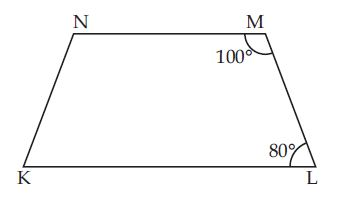The given figure KLMN is a trapezium, as its two sides KL and MN are parallel, because, sum of its adjacent angles $\angle$L and $\angle$M is 180$°$.

##### Question 41:

Find m$\angle$C in the figure if AB || DC.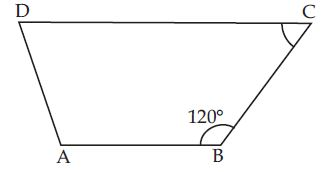It is given that, ABCD is a trapezium having
$\angle$B = 120$°$ and two of its sides AB and CD are
parallel.
$\therefore$ $\angle$B + $\angle$C = 180$°$
$⇒$ 120$°$ + $\angle$C = 180$°$
$⇒$ $\angle$C = 180$°$ – 120$°$ = 60$°$

##### Question 42:

Find the measure of $\angle$P and $\angle$S if SP || RQ in Fig. (If you find m$\angle$R, is there more than one method to find m$\angle$P?).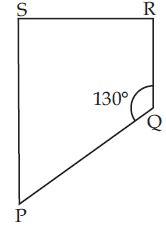It is given that, PQRS is a trapezium having $\angle$Q =
130$°$ and two of its sides PS and RQ are parallel.
$\therefore$ $\angle$P + $\angle$Q = 180$°$
$⇒$ $\angle$P + 130$°$ = 180$°$
$⇒$ $\angle$P = 50$°$
Also, $\angle$R and $\angle$S each have measure 90$°$.
We may find $\angle$P by one more method.
i.e., Sum of all the interior angles of a quadrilateral is 360$°$.
$\therefore$ $\angle$P + $\angle$Q + $\angle$R + $\angle$S = 360$°$
$⇒$ $\angle$P + 130$°$ + 90$°$ + 90$°$ = 360$°$
$⇒$ $\angle$P + 310$°$ = 360$°$
$⇒$ $\angle$P = 360$°$ – 310$°$
$⇒$ $\angle$P = 50$°$

##### Question 43:

Take a square sheet, say PQRS (Fig (i)). Fold along both the diagonals. Are their mid-point the same? Check if the angle at O is 90$°$ by using a set-square.
This verifies the property stated above.

Yes, their mid-point is the same and the angle at O is 90$°$.
We know that PQSR is a square whose diagonals meet at O.
PO = OR (Since, the square is a parallelogram)
By SSS congruency condition, we see that
$∆$POQ $\cong$ $∆$SOR
[$\therefore$ PQ = SR; PO = OR; SO
= OQ]
$\therefore$ m$\angle$POQ = m$\angle$SOR
These angles being a linear
pair, each is right angle.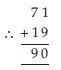##### Question 44:

All rectangles are squares.

FALSE

##### Question 45:

All rhombuses are parallelograms.

TRUE

##### Question 46:

All squares are rhombuses and also rectangles.

TRUE

##### Question 47:

All squares are not parallelograms.

FALSE

##### Question 48:

All kites are rhombuses.

FALSE

##### Question 49:

All rhombuses are kites.

TRUE

##### Question 50:

All parallelograms are trapeziums.

TRUE

##### Question 51:

All squares are trapeziums.

TRUE

##### Question 52:

Identify all the quadrilaterals that have:
(a) Four sides of equal length
(b) Four right angles

(a) Square and Rhombus
(b) Square and Rectangle

##### Question 53:

Explain how a square is:
(ii) a parallelogram
(iii) a rhombus
(iv) a rectangle

(i) Any four sided figure is called a quadrilateral and so is the square.
(ii) Opposite sides of a parallelogram are equal and parallel and so is in the square.
(iii) All the four sides of a rhombus are equal and so is in the square.
(iv) All the four angles of a rectangle are right angles and opposite sides are equal, same is the case with the square.

##### Question 54:

(i) bisect each other.
(ii) are perpendicular bisectors of each other.
(iii) are equal.

(i) Parallelogram, Rhombus, Square, Rectangle
(ii) Rhombus, Square.
(iii) Rectangle, Square.

##### Question 55:

Explain why a rectangle is a convex quadrilateral.

A rectangle is a convex quadrilateral, because no part of its diagonals lies in its exteriors.

##### Question 56:

ABC is a right-angled triangle and O is the midpoint of the side opposite to the right angle. Explain why O is equidistant from A, B and C. (The dotted lines are drawn additionally to help you).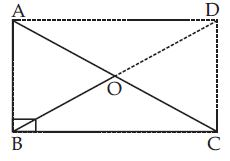$\therefore$ O is the mid-point of AC as well as BD.
$\therefore$ O is equidistant from A, B, C and D as well.